## SBT Solution Lesson 2: Equal triangles (C8 SBT Math 7 Horizons) – Math Book

Solving SBT Lesson 2: Equal triangles (C8 SBT Math 7 Horizons)
==========

### Solution 1 page 45 SBT Math 7 Creative horizon episode 2 – CTST

In Figure 12, find triangle equal to triangle ABH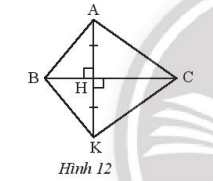Detailed instructions for solving Lesson 1

Solution method

Check the conditions of the two triangles ABH and KBH.

Detailed explanation

Consider triangle ABH and triangle KBH with:

General insurance

$$\widehat {AHB} = \widehat {KHB} = {90^o}$$

AB = KB

Derive: $$\Delta ABH = \Delta KBH(c – g – c)$$

–>

— *****

### Solution 2 page 45 SBT Math 7 Creative horizon episode 2 – CTST

Are the two triangles in figure 13a and 13b congruent? Why?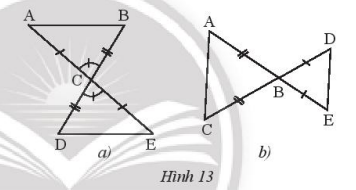Detailed instructions for solving Lesson 2

Solution method

Check for congruence cases of two congruent triangles.

Detailed explanation

* Figure 13a: Consider $$\Delta ACB$$ and $$\Delta ECB$$ with:

AC = EC

$$\widehat {ACB} = \widehat {EC{\rm{D}}}$$

BC = DC

Derive: $$\Delta ACB = \Delta EC{\rm{D}}(c – g – c)$$

* Figure 14a: Two triangles ABC and DBC are not congruent because two triangles ABC and DBC have no corresponding corresponding angle.

–>

— *****

### Solution 3 page 45 SBT Math 7 Creative horizon episode 2 – CTST

State more conditions for the two triangles in figure 14a, 14b to be congruent in the case of side – angle – side.’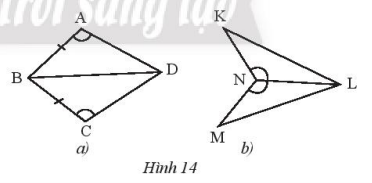Detailed instructions for solving Lesson 3

Solution method

Consider the conditions on two sides and the included angle to give reasonable conditions

Detailed explanation

* Figure 14a: Consider $$\Delta AB{\rm{D}}$$ and $$\Delta CB{\rm{D}}$$ with:

AB = BC

$$\widehat {BA{\rm{D}}} = \widehat {BC{\rm{D}}}$$

To $$\Delta AB{\rm{D}}$$ = $$\Delta CB{\rm{D}}$$(c – g – c) you need to add the condition AD = CD

* Figure 14b: Consider $$\Delta KNL$$ and $$\Delta MNL$$ with:

General capacity

$$\widehat {KNL} = \widehat {MNL}$$ ( c – g – c) then need to add the condition that KN = MN

–>

— *****

### Solution 4 page 45 SBT Math 7 Creative horizon episode 2 – CTST

Observe figure 15 and then change the sign ? with the appropriate triangle name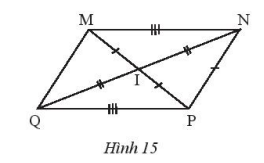a) $$\Delta MNI = \Delta ?$$

b) $$\Delta INM = \Delta ?$$

c) $$\Delta ? = \Delta QIP$$

Detailed instructions for solving Lesson 4

Solution method

Consider the conditions of congruence of two triangles

Detailed explanation

a) $$\Delta MNI = \Delta PQI$$( because there is MI = PI, MN = PQ, NI = QI)

b) $$\Delta INM = \Delta IQP$$ (because IN = IQ; NM = QP, IM = IP)

c) $$\Delta NIM = \Delta QIP$$ (because NI = QI, IM = IP, NM = QP)

–>

— *****

### Solve problems 5 pages 46 SBT Math 7 Creative horizon episode 2 – CTST

Given $$\Delta ABC = \Delta D{\rm{EF}}$$ and $$\widehat {{A^{}}} = {44^o}$$, EF = 7 cm, ED = 15 cm . Calculate the measure $$\widehat D$$ and the lengths BC, BA.

Detailed instructions for solving Lesson 5

Solution method

From two congruent triangles, the corresponding sides and angles are congruent.

Detailed explanation

We have $$\Delta ABC = \Delta D{\rm{EF}}$$ so that:

$$\widehat D = \widehat {{A^{}}} = {44^o}{,^{}}BC = {\rm{EF = 7(cm)}}{{\rm{,}} ^{}}BA = E{\rm{D}} = 15(cm)$$

–>

— *****

### Solve lesson 6 page 46 SBT Math 7 Creative horizon episode 2 – CTST

Are the pairs of triangles in Figure 16 congruent? If so, under what circumstances are they equal?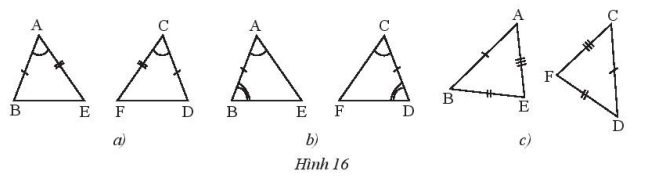Detailed instructions for solving Lesson 6

Solution method

Check the conditions according to the congruence cases of two triangles

Detailed explanation

a) $$\Delta A{\rm{E}}B = \Delta CF{\rm{D}}$$according to the case of alignment – ​​angle – side

b) $$\Delta ABE = \Delta C{\rm{D}}F$$ in the corner – side – angle case

c) $$\Delta ABE = \Delta C{\rm{D}}F$$in the edge-to-edge case

–>

— *****

### Solution 7 page 46 SBT Math 7 Creative horizon episode 2 – CTST

Let $$\Delta ABC = \Delta D{\rm{EF}}$$ and AB = 9 cm, AC = 7 cm, EF = 10 cm. Calculate the perimeter of triangle ABC.

Detailed instructions for solving Lesson 7

Solution method

From two congruent triangles, the corresponding sides and angles are congruent.

Detailed explanation

Since $$\Delta ABC = \Delta D{\rm{EF}}$$ BC = EF = 10 (cm)

Therefore, the perimeter of triangle ABC is: 9 + 7 + 10 = 26 (cm)

–>

— *****

### Solve problem 8 page 46 SBT Math 7 Creative horizon episode 2 – CTST

Given triangle ABC with AB = AC, take a point M on side BC such that BM = CM. Prove that triangles ABM and ACM are congruent.

Detailed instructions for solving Lesson 8

Solution method

Consider the angle conditions to see in which case two triangles are congruent?

Detailed explanation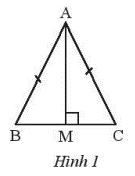Consider two triangles ABM and ACM with:

AB = AC

BM = CM (assumption)

AM is the common edge

Derive: $$\Delta ABM = \Delta ACM(c – c – c)$$

–>

— *****

### Solve problem 9 page 46 SBT Math 7 Creative horizon episode 2 – CTST

Given the angle xOy. Take two points A and B on the ray Ox such that OA < OB. Take two points C and D on the ray Oy such that OA = OC, OB = OD. Let M be the intersection of AD and CB. Prove that:

b) $$\Delta MAB = \Delta MC{\rm{D}}$$

Detailed instructions for solving Lesson 9

Solution method

Prove that two triangles have two corresponding sides congruent.

– Considering the conditions of two triangles MAB and MCD, in what cases are those two triangles congruent?

Detailed explanationa) Consider triangle AOD and triangle COB with:

OA = OC

$$\widehat O$$ common

OB = OD

Derive: $$\Delta AO{\rm{D}} = \Delta COB(c – g – c)$$

b) We have: $$\Delta AO{\rm{D}} = \Delta COB(c – g – c)$$(prove above)

Consider triangle MAB and triangle MCD with:

Thus: $$\widehat {MBA} = \widehat {M{\rm{D}}C};\widehat {MAB} = \widehat {MC{\rm{D}}}$$ equal)

AB = CD (because OA = OC, OB = OD)

Derive: $$\Delta MAB = \Delta MC{\rm{D(g – c – g)}}$$

–>

— *****